### Home > CALC > Chapter 4 > Lesson 4.3.2 > Problem4-110

4-110.
1. Write each of the following integral expressions as a single integral. Homework Help ✎

1.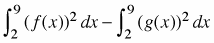2.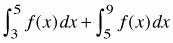3.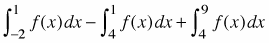4.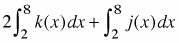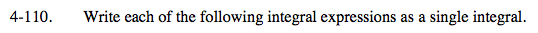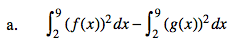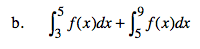Translation: The area between 3 and 5 plus the area between 5 and 9.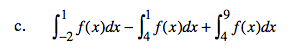$\int_{a}^{b}f(x)dx=\int_{a}^{b}-f(x)dx$

$\int_{-2}^{9}f(x)dx$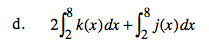Translation: TWO TIMES the area under k(x) on [2,8] + ONE TIME the area under k(x) on [2,8].